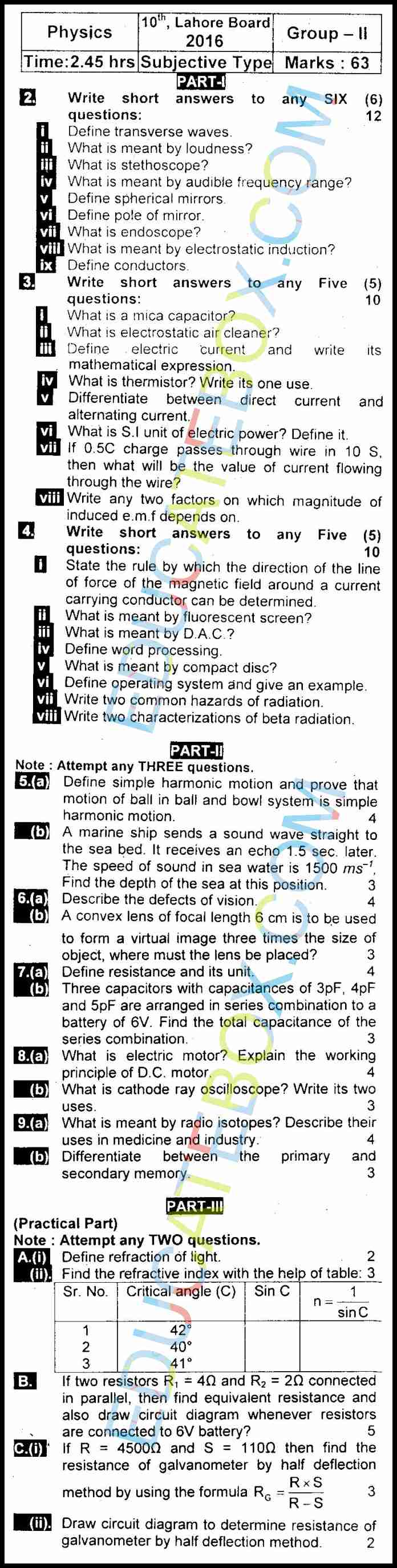# Past Paper 10th Physics Lahore 2016 Group 2 (English Medium)

0
257

## Past Paper Class 10 Physics Lahore Board 2016 OBJECTIVE Type Group 2 (English Medium)

Class 10th Physics (English Medium) past paper 2016, group 2 of Lahore board consists of two parts. First part is objective type and second part is subjective type.  Objective type consists of following important questions:

• If mass of the bob of a pendulum is increased by a factor of 3, the period of pendulum’s motion will ___.
• The characteristic of sound by which we can distinguish between two sounds of same loudness and pitch is called ____.
• The focal length (f) is related to radius of curvature (R) as ____.
• In series combination of capacitors, each capacitor will have sam ______
• A lamp connected to a 12V source, when it carries 2.5A current, power will ____.
• Term “e-mail” stands for Electronic mail and Example of primary memory is ROM.

## Past Paper Class 10 Physics Lahore Board 2016 SUBJECTIVE Type Group 2 (English Medium)

Some of the subjective questions are as follows:

• What is meant by audible frequency range?
• What is meant by electrostatic induction? What is conductor?
• Differentiate between direct current and alternating current.
• If 0.5C charge passes through wire in 10 S, then what will be the value of current flowing through the wire?
• Write any two factors on which magnitude of induced e.m.f depends on.
• State the rule by which the direction of the line of force of the magnetic field around a current carrying conductor can be determined.
• Define operating system and give an example.
• Define simple harmonic motion and prove that motion of ball in ball and bowl system is simple harmonic motion.
• A convex lens of focal length 6 cm is to be used to form a virtual image three times the size of object, where must the lens be placed?
• What is electric motor? Explain the working principle of D.C. motor.
• What is cathode ray oscilloscope? Write its two uses.
• What is meant by radio isotopes? Describe their uses in medicine and industry.